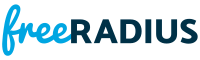rlm_eap_pwd.c File Reference
#include "rlm_eap_pwd.h"
#include "eap_pwd.h"Include dependency graph for rlm_eap_pwd.c:

Go to the source code of this file.

Macros

#define MPPE_KEY_LEN   32

#define MSK_EMSK_LEN   (2*MPPE_KEY_LEN)

Functions

static int _free_pwd_session (pwd_session_t *session)

static int CC_HINT (nonnull)

static int mod_detach (void *arg)

static int mod_instantiate (CONF_SECTION *cs, void **instance)

static int mod_process (void *arg, eap_session_t *eap_session)

static int send_pwd_request (pwd_session_t *session, eap_round_t *eap_round)

Variables

static CONF_PARSER pwd_module_config []

rlm_eap_module_t rlm_eap_pwd

Macro Definition Documentation

 #define MPPE_KEY_LEN   32

Definition at line 41 of file rlm_eap_pwd.c.

 #define MSK_EMSK_LEN   (2*MPPE_KEY_LEN)

Definition at line 42 of file rlm_eap_pwd.c.

Function Documentation

 static int _free_pwd_session ( pwd_session_t * session )
static

Definition at line 85 of file rlm_eap_pwd.c.Here is the caller graph for this function:

 static int CC_HINT ( nonnull )
static

Definition at line 171 of file rlm_eap_pwd.c.Here is the call graph for this function:

 static int mod_detach ( void * arg )
static

Definition at line 52 of file rlm_eap_pwd.c.

 static int mod_instantiate ( CONF_SECTION * cs, void ** instance )
static

Definition at line 63 of file rlm_eap_pwd.c.Here is the call graph for this function:

 static int mod_process ( void * arg, eap_session_t * eap_session )
static

Definition at line 268 of file rlm_eap_pwd.c.Here is the call graph for this function:Here is the caller graph for this function:

 static int send_pwd_request ( pwd_session_t * session, eap_round_t * eap_round )
static

Definition at line 101 of file rlm_eap_pwd.c.Here is the caller graph for this function:

Variable Documentation

 CONF_PARSER pwd_module_config[]
static
Initial value:
= {
{ FR_CONF_OFFSET("group", PW_TYPE_INTEGER, eap_pwd_t, group), .dflt = "19" },
{ FR_CONF_OFFSET("fragment_size", PW_TYPE_INTEGER, eap_pwd_t, fragment_size), .dflt = "1020" },
{ FR_CONF_OFFSET("server_id", PW_TYPE_STRING, eap_pwd_t, server_id) },
{ FR_CONF_OFFSET("virtual_server", PW_TYPE_STRING, eap_pwd_t, virtual_server) },
}
#define CONF_PARSER_TERMINATOR
Definition: conffile.h:289
32 Bit unsigned integer.
#define FR_CONF_OFFSET(_n, _t, _s, _f)
Definition: conffile.h:168
String of printable characters.

Definition at line 44 of file rlm_eap_pwd.c.

 rlm_eap_module_t rlm_eap_pwd
Initial value:
= {
.name = "eap_pwd",
.instantiate = mod_instantiate,
.session_init = mod_session_init,
.process = mod_process,
.detach = mod_detach
}
static int mod_instantiate(CONF_SECTION *cs, void **instance)
Definition: rlm_eap_pwd.c:63
static int mod_process(void *arg, eap_session_t *eap_session)
Definition: rlm_eap_pwd.c:268
static int mod_session_init(void *instance, eap_session_t *eap_session)
Definition: rlm_eap_gtc.c:89
static int mod_detach(void *arg)
Definition: rlm_eap_pwd.c:52

Definition at line 626 of file rlm_eap_pwd.c.